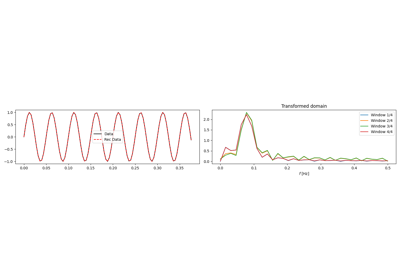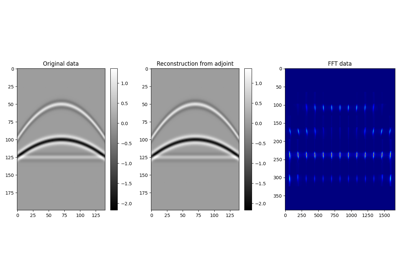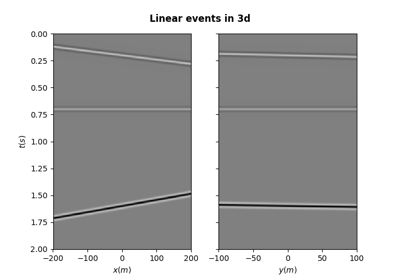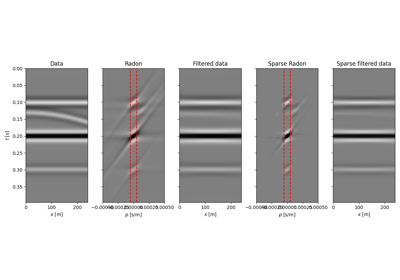# pylops.utils.seismicevents.parabolic2d#

pylops.utils.seismicevents.parabolic2d(x, t, t0, px, pxx, amp, wav)[source]#

Parabolic 2D events

Create 2d parabolic events given intercept time, slowness, curvature, and amplitude of each event

Parameters
xnumpy.ndarray

space axis

tnumpy.ndarray

time axis

t0

intercept time at $$x=0$$ of each parabolic event

px

slowness of each parabolic event

pxx

curvature of each parabolic event

amp

amplitude of each parabolic event

wavnumpy.ndarray

wavelet to be applied to data

Returns
dnumpy.ndarray

data without wavelet of size $$[n_x \times n_t]$$

dwavnumpy.ndarray

data with wavelet of size $$[n_x \times n_t]$$

Notes

Each event is created using the following relation:

$t_i(x) = t_{0,i} + p_{x,i} x + p_{xx,i} x^2$

## Examples using pylops.utils.seismicevents.parabolic2d#1D, 2D and 3D Sliding

1D, 2D and 3D SlidingPatching

PatchingSynthetic seismic

Synthetic seismic